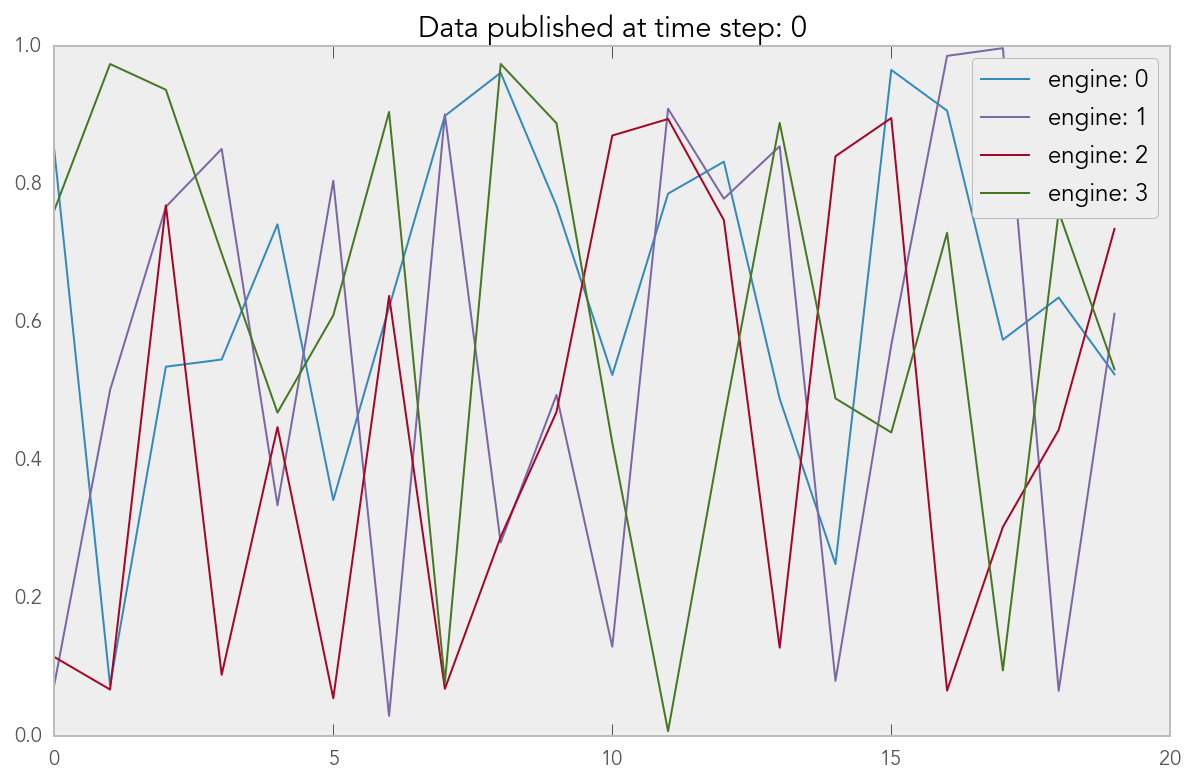# IPython’s Data Publication API#

IPython has an API that allows IPython Engines to publish data back to the Client. This Notebook shows how this API works.

## Setup#

We begin by enabling matplotlib plotting and creating a `Client` object to work with an IPython cluster.

```%matplotlib inline
```
```import ipyparallel as ipp
```
```c = ipp.Client()
dv = c[:]
dv.block = False
dv
```
```<DirectView [0, 1, 2, 3]>
```

## Simple publication#

Here is a simple Python function we are going to run on the Engines. This function uses `publish_data` to publish a simple Python dictionary when it is run.

```def publish_it():
from ipyparallel.datapub import publish_data
publish_data(dict(a='hi'))
```

We run the function on the Engines using `apply_async` and save the returned `AsyncResult` object:

```ar = dv.apply_async(publish_it)
```

The published data from each engine is then available under the `.data` attribute of the `AsyncResult` object.

```ar.data
```
```[{}, {}, {}, {}]
```

Each time `publish_data` is called, the `.data` attribute is updated with the most recently published data.

## Simulation loop#

In many cases, the Engines will be running a simulation loop and we will want to publish data at each time step of the simulation. To show how this works, we create a mock simulation function that iterates over a loop and publishes a NumPy array and loop variable at each time step. By inserting a call to `time.sleep(1)`, we ensure that new data will be published every second.

```def simulation_loop():
from ipyparallel.datapub import publish_data
import time
import numpy as np
for i in range(10):
publish_data(dict(a=np.random.rand(20), i=i))
time.sleep(1)
```

Again, we run the `simulation_loop` function in parallel using `apply_async` and save the returned `AsyncResult` object.

```ar = dv.apply_async(simulation_loop)
```

New data will be published by the Engines every second. Anytime we access `ar.data`, we will get the most recently published data.

```import matplotlib.pyplot as plt
```
```data = ar.data
for i, d in enumerate(data):
plt.plot(d['a'], label='engine: '+str(i))
plt.title('Data published at time step: ' + str(data['i']))
plt.legend()
```
```<matplotlib.legend.Legend at 0x105f75b00>
```